# Multiplying And Dividing Decimals Worksheet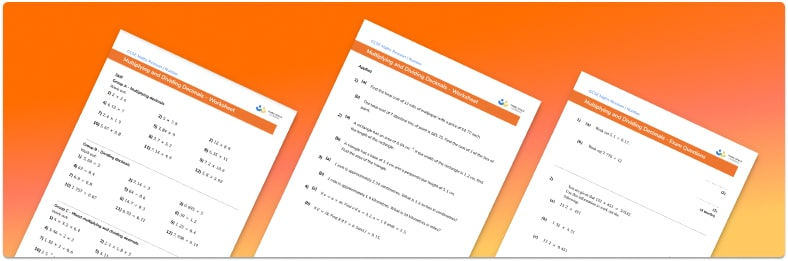• Section 1 of the multiplying and dividing decimals worksheet contains 36 skills-based multiplying and dividing decimals questions, in 3 groups to support differentiation
• Section 2 contains 4 applied ​​multiplying and dividing decimals questions with a mix of word problems and deeper problem solving questions
• Section 3 contains 4 foundation and higher level GCSE exam style multiplying and dividing decimals questions
• Answer keys and a mark scheme for all multiplying and dividing decimals questions are provided
• Questions follow variation theory with plenty of opportunities for students to work independently at their own level
• All questions created by fully qualified expert secondary maths teachers
• Suitable for GCSE maths revision for AQA, OCR and Edexcel exam boards

• This field is for validation purposes and should be left unchanged.

You can unsubscribe at any time (each email we send will contain an easy way to unsubscribe). To find out more about how we use your data, see our privacy policy.

### Multiplying and dividing decimals at a glance

A decimal number has a part that is not whole. We write the whole number part, followed by a decimal point, then tenths, hundredths, thousandths, and so on. It is important that students can recognise the decimal place value of each column.

When multiplying decimals, we use powers of ten to convert our calculation into a whole-number multiplication so that the standard multiplication algorithms can be used. For example, to work out 0.31.52, we would multiply the first number by 10 and the second number by 100, to get 3152. Once the whole number answer has been worked out (456), we reverse the multiplication by powers of 10 to get the answer to the decimal multiplication – so in this example, we divide our answer by 1000, to give 0.456.

The method for decimal division depends on what is being divided. For an integer divisor with a decimal dividend, we use the standard algorithm for short or long division for two or three-digit numbers, placing the decimal point in the quotient directly above its position in the dividend. If the divisor is a decimal, we write the division as a fraction and use the concept of equivalent fractions to convert to a whole-number calculation.

Further multiplication and division problems include unit conversions, money calculations, and using decimals in standard form calculations.

Looking forward, students can then progress to additional number worksheets, for example a or a.For more teaching and learning support on Number our GCSE maths lessons provide step by step support for all GCSE maths concepts.

## Related worksheets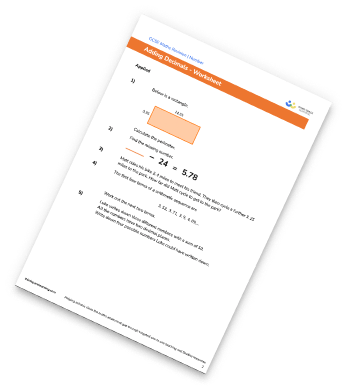Subtracting decimals worksheet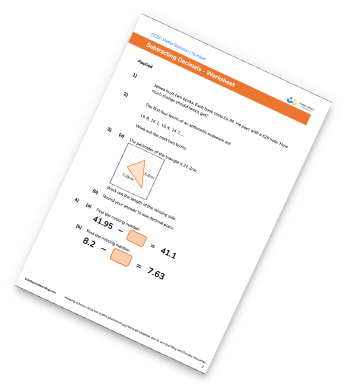Decimals Worksheets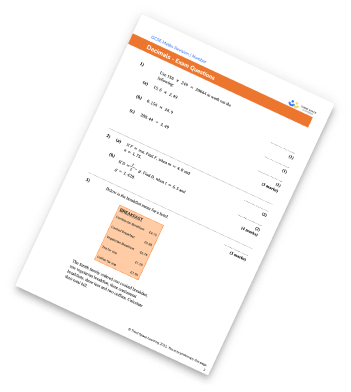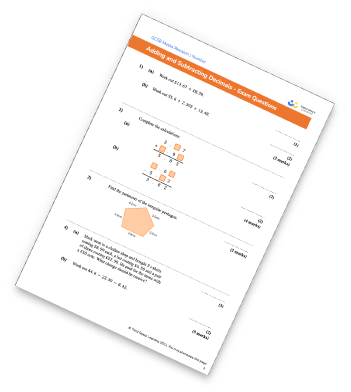## Do you have KS4 students who need more focused attention to succeed at GCSE?There will be students in your class who require individual attention to help them succeed in their maths GCSEs. In a class of 30, it’s not always easy to provide.

Help your students feel confident with exam-style questions and the strategies they’ll need to answer them correctly with our dedicated GCSE maths revision programme.

Lessons are selected to provide support where each student needs it most, and specially-trained GCSE maths tutors adapt the pitch and pace of each lesson. This ensures a personalised revision programme that raises grades and boosts confidence.

Find out more